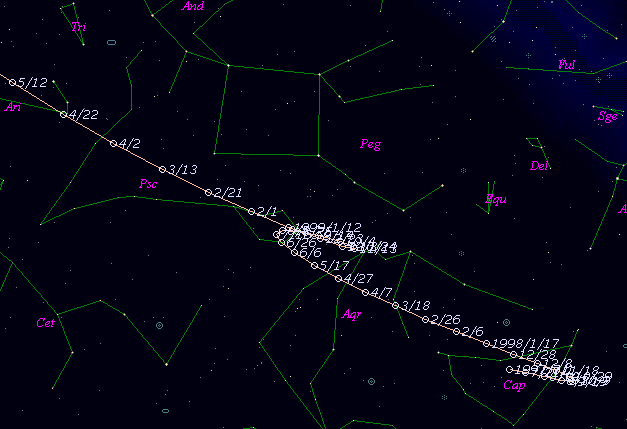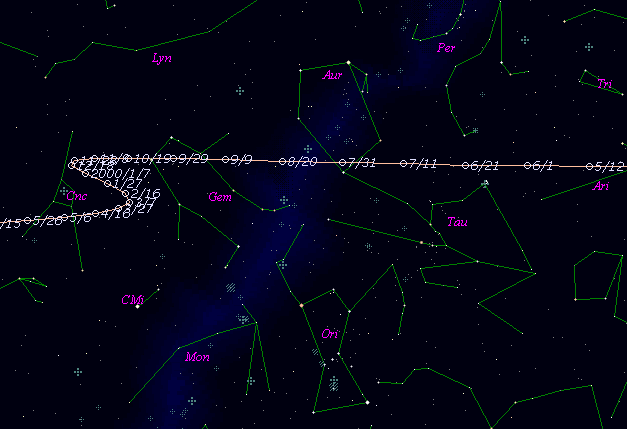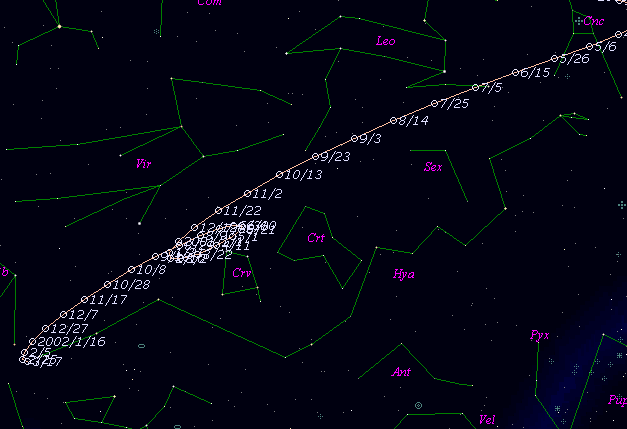# \$B%+!<%s%:(B-\$B%/%'!

59P/Kearns-Kwee (1999)###\$B###\$B50F;MWAG(B

```   The following improved orbital elements by Kenji Muraoka, are
from 153 observations  1981 to 1999, including 9 Planets, Moon
and 5 minor planets perturbations and non-gravitational effect
of style II.    The mean residual is +/- 0.85 arc seconds.

Epoch  =  1999 Sept. 19.0  TT      JDT = 2451440.5
T  =  1999 Sept. 16.33994      +/- 0.00084 (m.e.) TT
Peri. =  127.44633                +/- 0.00033
Node  =  313.03549                +/- 0.00014   (2000.0)
Incl. =    9.35211                +/- 0.00003
q  =    2.3391732              +/- 0.0000013 AU
e  =    0.4765474              +/- 0.0000003
a  =    4.4687396              +/- 0.0000008 AU
n  =    0.10433415             +/- 0.00000003
P  =    9.447                  +/- 0.0000026  years
A1  =   +0.793                  +/- 0.073
A2  =   -0.23426                +/- 0.00075
```

###\$B@1?^(B###\$B8wEYJQ2=(B

```        m1 = 7.0 + 5 log\$B&\$(B + 20.0 log r
```##### \$B50F;MWAG\$OB<2,7r<#;a\$N7W;;\$K\$h\$k\$b\$N\$G\$9!#(B \$B@1?^\$O(B StellaNavigator Ver.2.0 for Windows (\$B%"%9%H%m%"!<%D(B \$BJTCx(B / \$B%"%9%-!<=PHG6I4)(B) \$B\$G:n@.\$7\$?\$b\$N\$G\$9!#(B \$B8wEY%0%i%U\$O(BComet for Windows\$B\$G:n@.\$7\$?\$b\$N\$G\$9!#(B